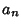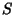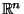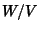## Hyperplane

Let,, ...,be Scalars not all equal to 0. Then the Setconsisting of all Vectorsinsuch thatis a Subspace ofcalled a hyperplane. More generally, a hyperplane is any co-dimension 1 vector Subspace of a Vector Space. Equivalently, a hyperplanein a Vector Spaceis any Subspace such thatis 1-dimensional. Equivalently, a hyperplane is the Kernel of any Nonzero linear Map from the Vector Space to the underlying Field.